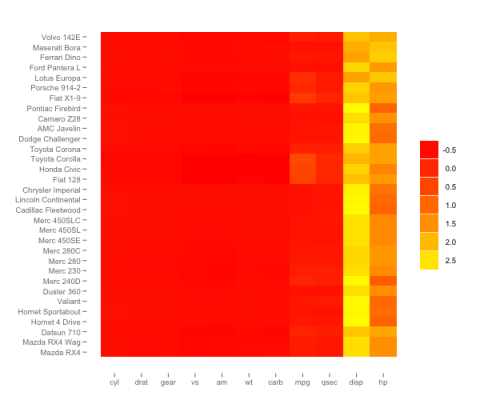Want to share your content on R-bloggers? click here if you have a blog, or here if you don't.

I hope the code here is fairly self-explanatory with the inset annotations. I feel this is just a bit ‘prettier’ than heatmap.2 and has for me the right balance of options and extensibility. I have also found it difficult to produce high quality plots with heatmap.2- whereas ggplots especially with RStudio assistance in resizing PNG turn out better IMHO.

```## m=matrix(data=sample(rnorm(100,mean=0,sd=2)), ncol=10)
## this function makes a graphically appealing heatmap (no dendrogram) using ggplot
## whilst it contains fewer options than gplots::heatmap.2 I prefer its style and flexibility

ggheat=function(m, rescaling='none', clustering='none', labCol=T, labRow=T, border=FALSE,
heatscale= c(low='blue',high='red'))
{
## the function can be be viewed as a two step process
## 1. using the rehape package and other funcs the data is clustered, scaled, and reshaped
## using simple options or by a user supplied function
## 2. with the now resahped data the plot, the chosen labels and plot style are built

require(reshape)
require(ggplot2)

## you can either scale by row or column not both!
## if you wish to scale by both or use a differen scale method then simply supply a scale
## function instead NB scale is a base funct

if(is.function(rescaling))
{
m=rescaling(m)
}
else
{
if(rescaling=='column')
m=scale(m, center=T)
if(rescaling=='row')
m=t(scale(t(m),center=T))
}

## I have supplied the default cluster and euclidean distance- and chose to cluster after scaling
## if you want a different distance/cluster method-- or to cluster and then scale
## then you can supply a custom function

if(is.function(clustering))
{
m=clustering(m)
}else
{
if(clustering=='row')
m=m[hclust(dist(m))\$order, ]
if(clustering=='column')
m=m[,hclust(dist(t(m)))\$order]
if(clustering=='both')
m=m[hclust(dist(m))\$order ,hclust(dist(t(m)))\$order]
}
## this is just reshaping into a ggplot format matrix and making a ggplot layer

rows=dim(m)
cols=dim(m)
melt.m=cbind(rowInd=rep(1:rows, times=cols), colInd=rep(1:cols, each=rows) ,melt(m))
g=ggplot(data=melt.m)

## add the heat tiles with or without a white border for clarity

if(border==TRUE)
g2=g+geom_rect(aes(xmin=colInd-1,xmax=colInd,ymin=rowInd-1,ymax=rowInd, fill=value),colour='white')
if(border==FALSE)
g2=g+geom_rect(aes(xmin=colInd-1,xmax=colInd,ymin=rowInd-1,ymax=rowInd, fill=value))

## add axis labels either supplied or from the colnames rownames of the matrix

if(labCol==T)
g2=g2+scale_x_continuous(breaks=(1:cols)-0.5, labels=colnames(m))
if(labCol==F)
g2=g2+scale_x_continuous(breaks=(1:cols)-0.5, labels=rep('',cols))

if(labRow==T)
g2=g2+scale_y_continuous(breaks=(1:rows)-0.5, labels=rownames(m))
if(labRow==F)
g2=g2+scale_y_continuous(breaks=(1:rows)-0.5, labels=rep('',rows))

## get rid of grey panel background and gridlines

g2=g2+opts(panel.grid.minor=theme_line(colour=NA), panel.grid.major=theme_line(colour=NA),
panel.background=theme_rect(fill=NA, colour=NA))

## finally add the fill colour ramp of your choice (default is blue to red)-- and return
return(g2+scale_fill_continuous("", heatscale, heatscale))

}

## NB because ggheat returns an ordinary ggplot you can add ggplot tweaks post-production e.g.
## data(mtcars)
## x= as.matrix(mtcars)
## ggheat(x, clustCol=T)+ opts(panel.background=theme_rect(fill='pink'))```

Here is quick example with the same colourscheme as gplots::heatmap.2

```data(mtcars)
x=as.matrix(mtcars)
ggheat(x, clustering='column', rescaling='row', heatscale=c(low='red', high='yellow'))```